Start typing, then use the up and down arrows to select an option from the list.# Half-Life Example 3

Jules Bruno
99views
The half life of a certain reaction with second order was found to be .45 seconds. What was the initial concentration of the reactant? If the slope of the straight line for this reaction is 3.5 times 10 to the -3. Alright, so they tell us it's second order which means that half life equals one over K. Times initial concentration here we need to solve for initial concentration. So what we do is we multiply both sides by K. Times initial concentration which becomes K. Times initial concentration times half life equals one, divide both sides. Now by K. And half life. And we've isolated our initial concentration. So initial concentration equals one over your rate constant K. Times your half life. Alright, so now knowing this let's solve so initial concentration equals one over. We're told that our Half life is .45 seconds. But how do we figure out? Kay remember when we dealt with the integrated weight loss when it comes to K. It's equal to the slope of a straight line. So them telling us the slope of the straight line is them really telling us what K. Is. So okay here is this value. So we plug in 3.5 times 10 to the -3. Doing that gives us our initial concentration which is 6:34.92 molars. Since both of our numbers have two sig figs, this comes out to 630 moller. So this would be our initial concentration of our reactant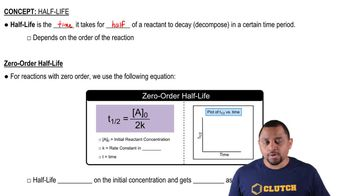02:17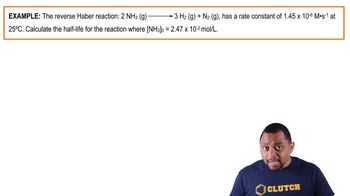01:19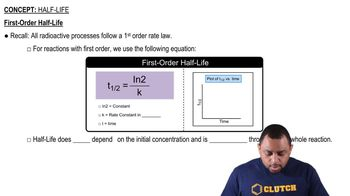01:49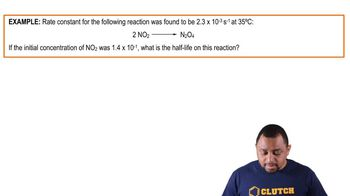01:18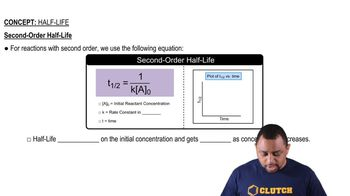01:44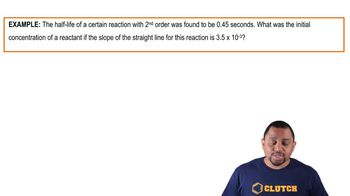01:52